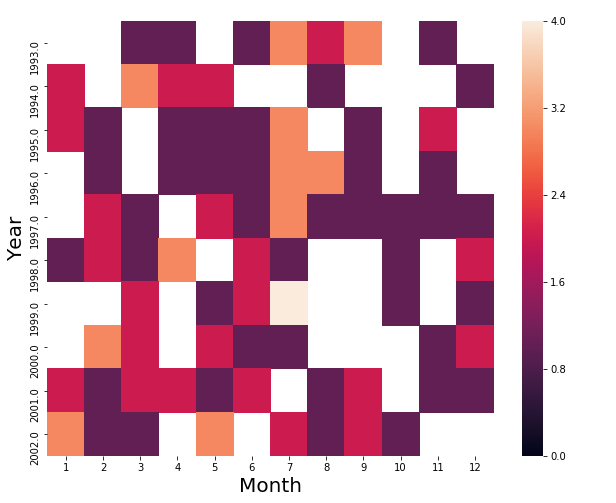﻿ Pandas Datetime: Create a heatmap for comparison of the top 10 years in which the UFO was sighted vs each Month - w3resource

# Pandas Datetime: Create a heatmap for comparison of the top 10 years in which the UFO was sighted vs each Month

## Pandas Datetime: Exercise-24 with Solution

Write a Pandas program to create a heatmap (rectangular data as a color-encoded matrix) for comparison of the top 10 years in which the UFO was sighted vs each Month.

Sample Solution:

Python Code:

``````import pandas as pd
import matplotlib.pyplot as plt
import seaborn as sns
#Source: https://bit.ly/1l9yjm9
df['Date_time'] = df['Date_time'].astype('datetime64[ns]')
def is_top_years(year):
if year in most_sightings_years.index:
return year
month_vs_year = df.pivot_table(columns=df['Date_time'].dt.month,index=df['Date_time'].dt.year.apply(is_top_years),aggfunc='count',values='city')
month_vs_year.columns = month_vs_year.columns.astype(int)
print("\nHeatmap for comparison of the top 10 years in which the UFO was sighted vs each month:")
plt.figure(figsize=(10,8))
ax = sns.heatmap(month_vs_year, vmin=0, vmax=4)
ax.set_xlabel('Month').set_size(20)
ax.set_ylabel('Year').set_size(20)
``````

Sample Output:

```Heatmap for comparison of the top 10 years in which the UFO was sighted vs each month:```

Python Code Editor:

Have another way to solve this solution? Contribute your code (and comments) through Disqus.

What is the difficulty level of this exercise?

﻿

## Python: Tips of the Day

F strings:

It is a common practice to add variables inside strings. F strings are by far the coolest way of doing it. To appreciate the f strings more, let's first perform the operation with the format function.

```name = 'Owen'
age = 25
print("{} is {} years old".format(name, age))
```

Output:

```Owen is 25 years old
```

We specify the variables that go inside the curly braces by using the format function at the end. F strings allow for specifying the variables inside the string.

```name = 'Owen'
age = 25
print(f"{name} is {age} years old")
```

Output:

```Owen is 25 years old
```

F strings are easier to follow and type. Moreover, they make the code more readable.

```A, B, C = {2, 4, 6}
print(A, B, C)
A, B, C = ['p', 'q', 'r']
print(A, B, C)
```

Output:

```2 4 6
p q r
```